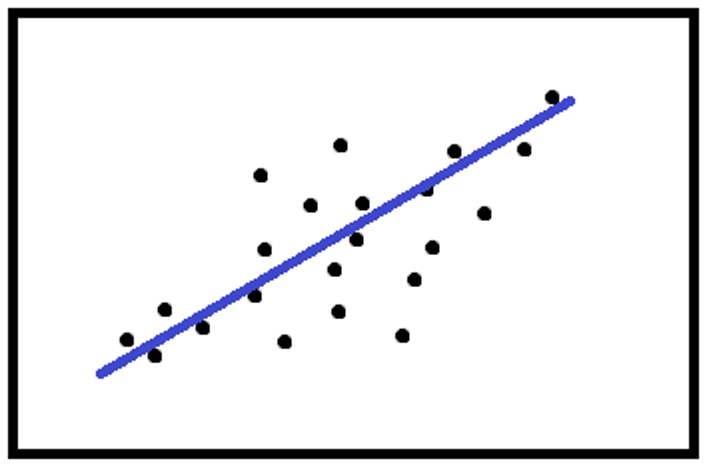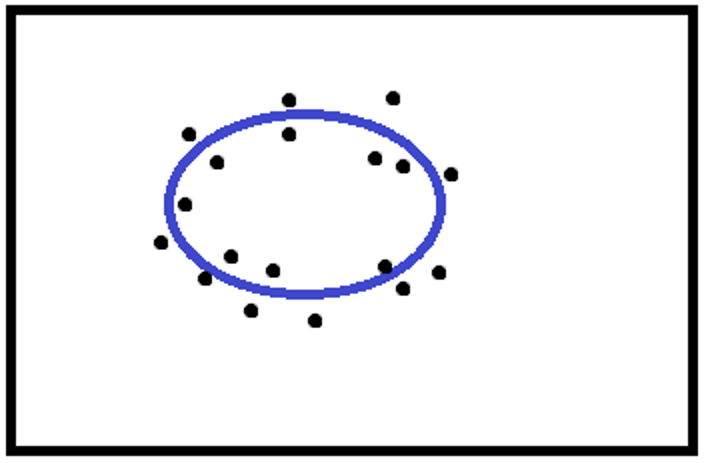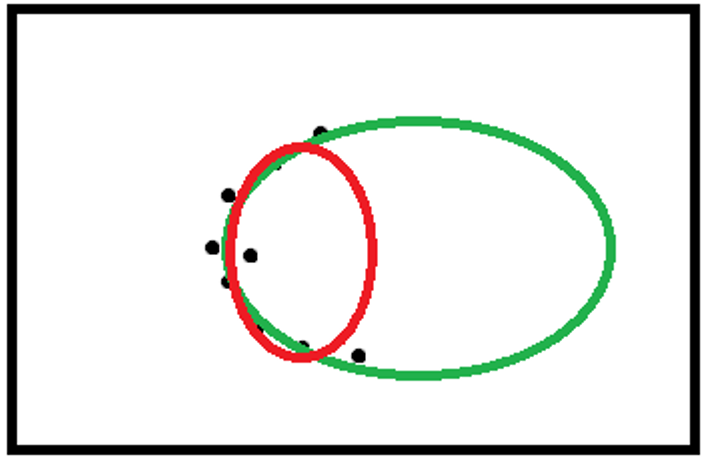## Shape fitting

When the outlines have been extracted, the geometrical primitives must be extracted from them.

The main ones are:

• Fitting lines
• Fitting circles
• Fitting ellipses

Linear and circular fitting

In both cases, the most commonly used algorithm is minimization, with the least-squares of the distance of the data points from the line or from the circumference.

The calculation can be made with the same weight for each pointOr, the weight can depend on the distance between the points and the calculated line or circumference.There are many functions to calculate the weights, the main ones being:

• Huber
w(d) {(1,dlec),(c/d,c<d):}

Where c is a value chosen by the user, d is the distance of the point of the primitive.

• Tukey
w(d) {((1-d^2/c^2)^2,dlec),(0,c<d):}

normally the value of c is set at twice the standard deviation of the distribution of the distances.

The difference between Huber’s and Tukey’s functions is that in the former, the weights have a unit value for d less than or equal to c, and the function never reaches zero, while in Tukey the weights always have different values for d less than or equal to c, and zero elsewhere.

Sometimes, the RANSAC algorithm is used to build a robust fit.

Elliptical fitting

The most commonly used algorithm is an algebraic method, called the Fitzgibbon method.

The elliptical fitting poses a few problems if the points only belong to certain parts of the ellipse

Examples:

• Correct fit• Ambiguous fitThe algorithm outcome may be the red ellipse, though the green ellipse is the correct one.

Next →